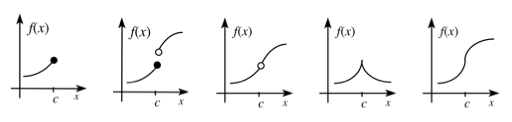### Home > CALC3RD > Chapter Ch5 > Lesson 5.4.1 > Problem5-146

5-146.

Multiple Choice: Let $f$ be the function given by $f(x)=|x|$. Which of the following statements about $f$ are true?

 I. $f$ is continuous at $x = 0$. II. $f$ is differentiable at $x = 0$. III. $f$ has an absolute minimum at $x = 0$.

Examples of functions that are not differentiable at $x = c$. Notice that some of them are still continuous at $x = c$.The Three Conditions of Continuity

$1. \lim \limits_{x \rightarrow a} f(x) \text{ exists} \\ 2. f(a) \text{ exists}\\ 3. \lim \limits_{x \rightarrow a} f(x) =f(a)$

 A. I only B. II only C. III only D. I and III E. II and III